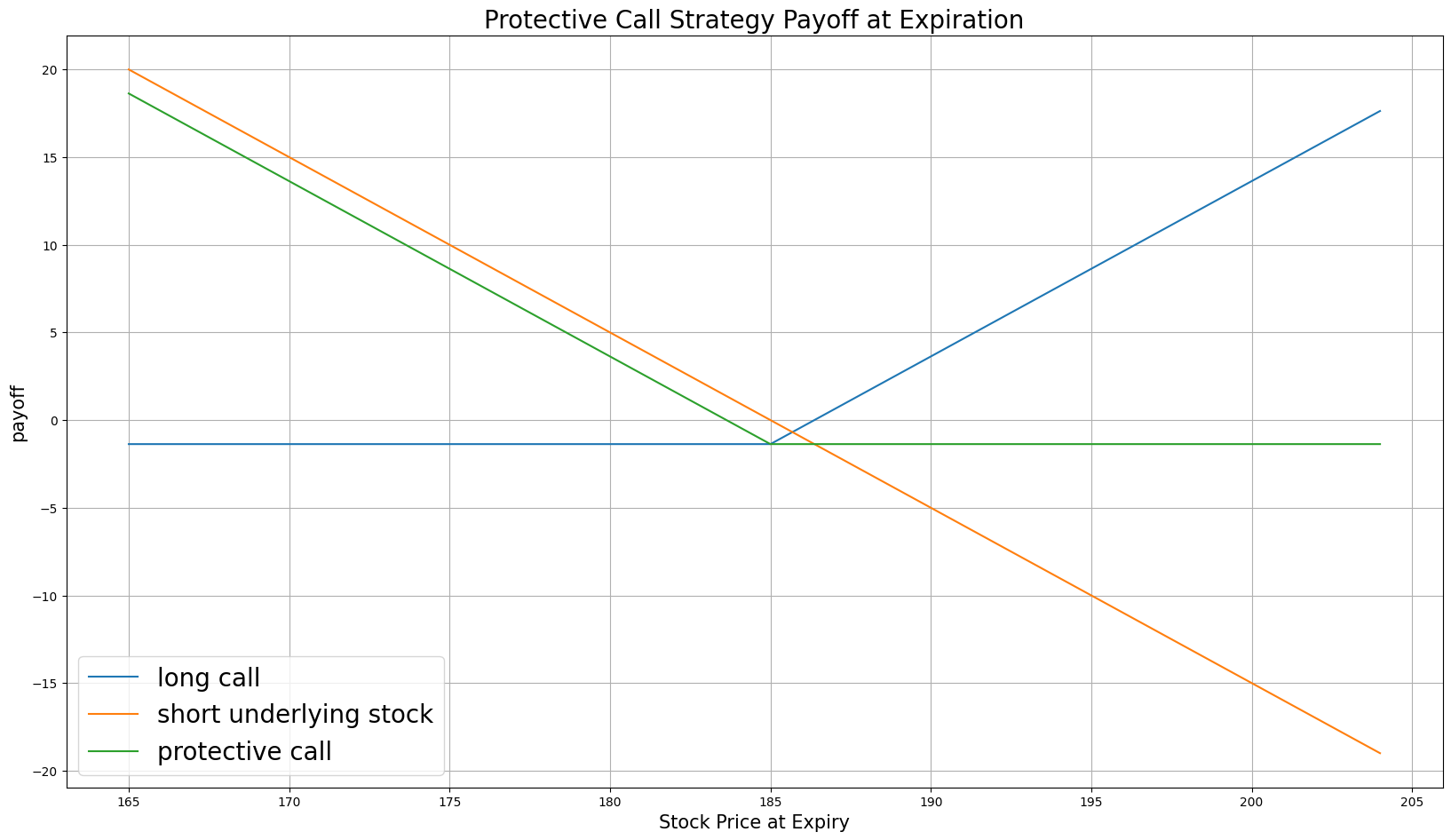# Option Strategies

## Protective Call

### Introduction

A Protective Call consists of a short position in a stock and a long position in a call Option for the same amount of stock. Protective calls aim to hedge the short position of a stock with a long ATM or slightly OTM call Option. At any time for American Options or at expiration for European Options, if the stock moves below the strike price, the Option contract becomes worthless but the short position acquires an unrealized gain. If the underlying price moves above the strike, you can exercise the Options contract and receive the underlying Equity, which closes your short position.

### Implementation

Follow these steps to implement the protective call strategy:

1. In the Initialize method, set the start date, end date, cash, and Options universe.
2. private Symbol _call, _symbol;

public override void Initialize()
{
SetStartDate(2014, 1, 1);
SetEndDate(2014, 3, 1);
SetCash(100000);

_symbol = option.Symbol;
option.SetFilter(-3, 3, 0, 31);
}
def Initialize(self) -> None:
self.SetStartDate(2014, 1, 1)
self.SetEndDate(2014, 3, 1)
self.SetCash(100000)

self.symbol = option.Symbol
self.call = None

option.SetFilter(-3, 3, 0, 31)
3. In the OnData method, select the Option contract.
4. public override void OnData(Slice slice)
{
if (_call != null && Portfolio[_call].Invested) return;

if (!slice.OptionChains.TryGetValue(_symbol, out var chain)) return;

// Find ATM call with the farthest expiry
var expiry = chain.Max(x => x.Expiry);
var atmCall = chain
.Where(x => x.Right == OptionRight.Call && x.Expiry == expiry)
.OrderBy(x => Math.Abs(x.Strike - chain.Underlying.Price))
.FirstOrDefault();
def OnData(self, slice: Slice) -> None:
if self.call and self.Portfolio[self.call].Invested:
return

chain = slice.OptionChains.get(self.symbol)
if not chain:
return

# Find ATM call with the farthest expiry
expiry = max([x.Expiry for x in chain])
call_contracts = sorted([x for x in chain
if x.Right == OptionRight.Call and x.Expiry == expiry],
key=lambda x: abs(chain.Underlying.Price - x.Strike))

if not call_contracts:
return

atm_call = call_contracts
5. In the OnData method, call the OptionStrategies.ProtectiveCall method and then submit the order.
6. var protectiveCall = OptionStrategies.ProtectiveCall(_symbol, atmCall.Strike, expiry);

_call = atmCall.Symbol;
protective_call = OptionStrategies.ProtectiveCall(self.symbol, atm_call.Strike, expiry)

self.call = atm_call.Symbol

Option strategies synchronously execute by default. To asynchronously execute Option strategies, set the asynchronous argument to Falsefalse. You can also provide a tag and order properties to the Buy method.

Buy(optionStrategy, quantity, asynchronous, tag, orderProperties);

self.Buy(option_strategy, quantity, asynchronous, tag, order_properties)


### Strategy Payoff

The payoff of the strategy is

$$\begin{array}{rcll} C^{K}_T & = & (S_T - K)^{+}\\ P_T & = & (S_0 - S_T + C^{K}_T - C^{K}_0)\times m - fee \end{array}$$ $$\begin{array}{rcll} \textrm{where} & C^{K}_T & = & \textrm{Call value at time T}\\ & S_T & = & \textrm{Underlying asset price at time T}\\ & K & = & \textrm{Call strike price}\\ & P_T & = & \textrm{Payout total at time T}\\ & S_0 & = & \textrm{Underlying asset price when the trade opened}\\ & C^{K}_0 & = & \textrm{Call price when the trade opened (credit received)}\\ & m & = & \textrm{Contract multiplier}\\ & T & = & \textrm{Time of expiration} \end{array}$$

The following chart shows the payoff at expiration:The maximum profit is $S_0 - C^{K}_0$, which occurs when the underlying price is $0$.

The maximum loss is $S_0 - K - C^{K}_0$, which occurs when the underlying price is above the strike price.

### Example

The following table shows the price details of the assets in the algorithm:

AssetPrice ($)Strike ($)
Call3.50185.00
Underlying Equity at start of the trade186.94-
Underlying Equity at expiration190.01-

Therefore, the payoff is

$$\begin{array}{rcll} C^{K}_T & = & (S_T - K)^{+}\\ & = & (190.01 - 185)^{+}\\ & = & 5.01\\ P_T & = & (S_0 - S_T + C^{K}_T - C^{K}_0)\times m - fee\\ & = & (186.94 - 190.01 + 5.01 - 3.50)\times m - fee\\ & = & -1.56 \times 100 - 2\\ & = & -158 \end{array}$$

So, the strategy losses \$158.

The following algorithm implements a protective call Option strategy:

You can also see our Videos. You can also get in touch with us via Discord.